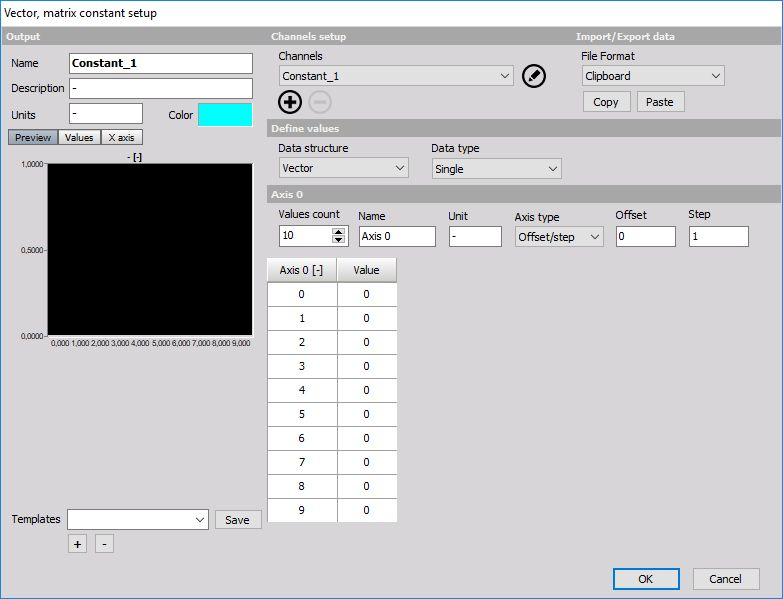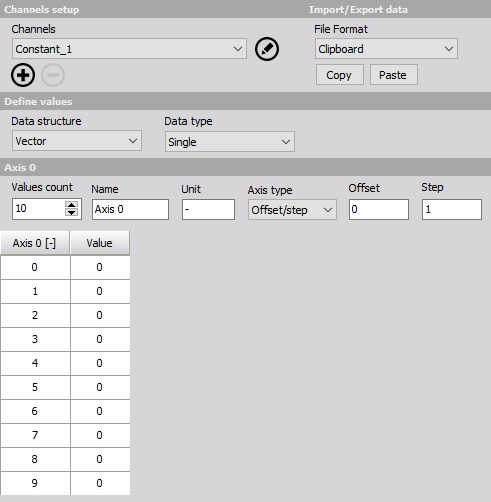# Vector matrix constant

When you press the Setup button or open a new math Matrix constant line, the following setup window will open:The constant can define a set of values which will be as a result an array channel. We can add or remove points by increasing or decreasing Values count number and we can also Copy / Paste values from/to Excel.

The resulting constant can be for example used when we want to multiply an array from FFT math with some filter parameters defined in the constant.